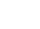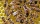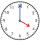# Fraction calculator

The calculator performs basic and advanced operations with fractions, expressions with fractions combined with integers, decimals, and mixed numbers. It also shows detailed step-by-step information about the fraction calculation procedure. Solve problems with two, three, or more fractions and numbers in one expression.

## Result:

### 21/4 + 3/4 = 3/1 = 3

Spelled result in words is three.

### How do you solve fractions step by step?

1. Conversion a mixed number 2 1/4 to a improper fraction: 2 1/4 = 2 1/4 = 2 · 4 + 1/4 = 8 + 1/4 = 9/4

To find new numerator:
a) Multiply the whole number 2 by the denominator 4. Whole number 2 equally 2 * 4/4 = 8/4
b) Add the answer from previous step 8 to the numerator 1. New numerator is 8 + 1 = 9
c) Write a previous answer (new numerator 9) over the denominator 4.

Two and one quarter is nine quarters
2. Add: 9/4 + 3/4 = 9 + 3/4 = 12/4 = 4 · 3/4 · 1 = 3
For adding, subtracting, and comparing fractions, it is suitable to adjust both fractions to a common (equal, identical) denominator. The common denominator you can calculate as the least common multiple of both denominators - LCM(4, 4) = 4. In practice, it is enough to find the common denominator (not necessarily the lowest) by multiplying the denominators: 4 × 4 = 16. In the next intermediate step, , cancel by a common factor of 4 gives 3/1.
In words - nine quarters plus three quarters = three.

#### Rules for expressions with fractions:

Fractions - use the slash “/” between the numerator and denominator, i.e., for five-hundredths, enter 5/100. If you are using mixed numbers, be sure to leave a single space between the whole and fraction part.
The slash separates the numerator (number above a fraction line) and denominator (number below).

Mixed numerals (mixed fractions or mixed numbers) write as non-zero integer separated by one space and fraction i.e., 1 2/3 (having the same sign). An example of a negative mixed fraction: -5 1/2.
Because slash is both signs for fraction line and division, we recommended use colon (:) as the operator of division fractions i.e., 1/2 : 3.

Decimals (decimal numbers) enter with a decimal point . and they are automatically converted to fractions - i.e. 1.45.

The colon : and slash / is the symbol of division. Can be used to divide mixed numbers 1 2/3 : 4 3/8 or can be used for write complex fractions i.e. 1/2 : 1/3.
An asterisk * or × is the symbol for multiplication.
Plus + is addition, minus sign - is subtraction and ()[] is mathematical parentheses.
The exponentiation/power symbol is ^ - for example: (7/8-4/5)^2 = (7/8-4/5)2

#### Examples:

adding fractions: 2/4 + 3/4
subtracting fractions: 2/3 - 1/2
multiplying fractions: 7/8 * 3/9
dividing Fractions: 1/2 : 3/4
exponentiation of fraction: 3/5^3
fractional exponents: 16 ^ 1/2
adding fractions and mixed numbers: 8/5 + 6 2/7
dividing integer and fraction: 5 ÷ 1/2
complex fractions: 5/8 : 2 2/3
decimal to fraction: 0.625
Fraction to Decimal: 1/4
Fraction to Percent: 1/8 %
comparing fractions: 1/4 2/3
multiplying a fraction by a whole number: 6 * 3/4
square root of a fraction: sqrt(1/16)
reducing or simplifying the fraction (simplification) - dividing the numerator and denominator of a fraction by the same non-zero number - equivalent fraction: 4/22
expression with brackets: 1/3 * (1/2 - 3 3/8)
compound fraction: 3/4 of 5/7
fractions multiple: 2/3 of 3/5
divide to find the quotient: 3/5 ÷ 2/3

The calculator follows well-known rules for order of operations. The most common mnemonics for remembering this order of operations are:
PEMDAS - Parentheses, Exponents, Multiplication, Division, Addition, Subtraction.
BEDMAS - Brackets, Exponents, Division, Multiplication, Addition, Subtraction
BODMAS - Brackets, Of or Order, Division, Multiplication, Addition, Subtraction.
GEMDAS - Grouping Symbols - brackets (){}, Exponents, Multiplication, Division, Addition, Subtraction.
Be careful, always do multiplication and division before addition and subtraction. Some operators (+ and -) and (* and /) has the same priority and then must evaluate from left to right.

## Fractions in word problems:

• Integer add to fraction7 is added to the sum of 4/5 and 6/7
• ExpressionsLet k represent an unknown number, express the following expressions: 1. The sum of the number n and two 2. The quotient of the numbers n and nine 3. Twice the number n 4. The difference between nine and the number n 5. Nine less than the number n
• ChestnutsThree divisions of nature protectors participated in the collection of chestnut trees.1. the division harvested 1250 kg, the 2nd division by a fifth more than the 1st division and the 3rd division by a sixth more than the second division. How many tons of
• Lengths of the poolMiguel swam 6 lengths of the pool. Mat swam 3 times as far as Miguel. Lionel swam 1/3 as far as Miguel. How many lengths did Mat swim?
• Ali boughtAli bought 5/6 litre of milk. He drank 1/2 litre and his brother drank 1/6 litre. How much litre of milk left?
• Algebra problemThis is algebra. Let n represent an unknown number. 1. Eight more than the number n 2. Three times the number n 3. The product of the number n and eight 4. Three less than the number n 5. Three decreased by the number n
• Math homeworkIt took Jose two-thirds of an hour to complete his math homework on Monday, three-fourths of an hour on Tuesday, any two- fifths of an hour on Wednesday. How many hours did it take Jose to complete his homework altogether?
• A 14.5-gallonA 14.5-gallon gasoline tank is 3/4 full. How many gallons will it take to fill the tank? Write your answer as a mixed number.
• Sum three fractionsWork out the sum of 1/4, 1/5 and 3/10.
• NotebooksLiza, a store owner, buys 560 notebooks. He sold 3/8 of the notebook, and then she adds the stock of a notebook of 1/4 of the number of notebooks she bought. What is the total number of notebooks she purchased?
• Sunny windowsillWe place a cup of water on a sunny windowsill. After the first day, 3/2 centimeters of water evaporated. After the second day, another 4/3 centimeters of water evaporated. How much total water, in centimeters, evaporated from the cup after the first two d
• Honey 2The baker has 3/4 liter of honey and decided to bake a few honeycombs and biscuits from it. 1/6 liter of honey was needed for honeycombs and 1/4 liter for biscuits. How much honey did she have left after baking honeycombs and biscuits?
• Bus vs trainMilada took the bus and the journey took 55 minutes. Jarmila was 1h 20 min by train. They arrived in Prague at the same time 10h45 min. At what time did each have to go out?